×#### Thank you for registering.

One of our academic counsellors will contact you within 1 working day.

Click to Chat

1800-1023-196

+91-120-4616500

CART 0

• 0

MY CART (5)

Use Coupon: CART20 and get 20% off on all online Study Material

ITEM
DETAILS
MRP
DISCOUNT
FINAL PRICE
Total Price: Rs.

There are no items in this cart.
Continue Shopping• Complete JEE Main/Advanced Course and Test Series
• OFFERED PRICE: Rs. 15,900
• View Details

```Chapter 7: Statistics Exercise – 7.3

Question: 1

The following table gives the distribution of total household expenditure (in rupees) of manual workers in a city.

Expenditure (in rupees) (x)
Frequency (fi)
Expenditure (in rupees) (xi)
Frequency (fi)

100 – 150
24
300 – 350
30

150 – 200
40
350 – 400
22

200 – 250
33
400 – 450
16

250 – 300
28
450 – 500
7

Find the average expenditure (in rupees) per household

Solution:

Let the assumed mean (A) = 275

Class interval
Mid value (xi)
di = xi – 275
ui = (xi - 275)/50
Frequency fi
fiui

100 – 150
125
-150
-3
24
-12

150 – 200
175
-100
-2
40
-80

200 – 250
225
-50
-1
33
-33

250 – 300
275
0
0
28
0

300 – 350
325
50
1
30
30

350 – 400
375
100
2
22
44

400 – 450
425
150
3
16
48

450 – 500
475
200
4
7
28

N = 200
Sum = -35

We have A = 275, h = 50
Mean = A + h * sum/N
= 275 + 50 * - 35/200
= 275 – 8.75
= 266.25

Question: 2

A survey was conducted by a group of students as a part of their environmental awareness program, in which they collected the following data regarding the number of plants in 200 houses in a locality. Find the mean number of plants per house.

Number of Number of plants:
0 - 2
2 – 4
4 – 6
6 – 8
8 – 10
10 – 12
12 – 14

Number of houses:

1
2
5
6
2
3

Which method did you use for finding the mean, and why?

Solution:

Let us find class marks (xi­) = (upper class limit + lower class limit)/2. Now we may compute xi and fixi as following.

Number of plants
Number of house (fi)
xi
Fixi

0 - 2
1
1
1

2 – 4
2
3
6

4 – 6
1
5
5

6 – 8
5
7
35

8 – 10
6
9
54

10 – 12
2
11
22

12 – 14
3
13
39

Total
N = 20

Sum = 162

From the table we may observe that N = 20, Sum = 162So mean number of plants per house is 8.1. We have used for the direct method values Xi and fi are very small

Question: 3

Consider the following distribution of daily wages of workers of a factory

Daily wages (in Rs)
100 - 120
120 - 140
140 - 160
160 - 180
180 - 200

Number of workers:
12
16
8
6
10

Find the mean daily wages of the workers of the factory by using an appropriate method.

Solution:

Let the assume mean (A) = 150

Class interval
Mid value xi
di = xi - 150
ui = (xi - 150)/20
Frequency fi
Fiui

100 – 120
110
-40
-2
12
-24

120 – 140
130
-20
-1
14
-14

140 – 160
150
0
0
8
0

160 – 180
170
20
1
6
6

180 – 200
190
40
2
10
20

N = 50
Sum = -12

We have N = 50, h = 20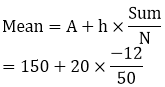= 150 - 4.8
= 145.2

Question: 4

Thirty women were examined in a hospital by a doctor and the number of heart beats per minute recorded and summarized as follows. Find the mean heart beats per minute for these women, choosing a suitable method. Number of heart

Beats Per minute:
65 - 68
68 - 71
71 - 74
74 - 77
77 - 80
80 - 83
83 - 86

Number of women:
2
4
3
8
7
4
2

Solution:

We may find marks of each interval (xi) by using the relation (xi¬) = (upper class limit + lower class limit)/2 Class size of this data = 3 Now talking 75.5 as assumed mean (a) We may calculate di, ui, fiui as following

Number of heart beats per minute
Number of women (xi)
xi
di = xi – 75.5
ui = (xi - 755)/h
fiui

65-68
2
66.5
-9
-3
-6

68-71
9
69.5
-6
-2
-8

71-74
3
72.5
-3
-1
-3

74-77
8
75.5
0
0
0

77-80
7
78.5
3
1
7

80-83
4
81.5
6
2
8

83-86
2
84.5
9
3
6

N = 30

Sum = 4

Now we may observe from table that N = 30, sum = 4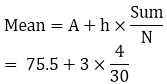= 75.5 + 0.4
= 75.9
So mean heart beats per minute for those women are 75.9 beats per minute

Question: 5

Find the mean of each of the following frequency distributions: (5 - 14)

Class interval:
0 - 6
6 – 12
12 – 18
18 – 24
24 – 30

Frequency:
6
8
10
9
7

Solution:

Let us assume mean be 15

Class interval
Mid - value
di = xi – 15
ui = (xi – 15)/6
fi
fiui

0 - 6
3
-12
-2
6
-12

12-Jun
9
-6
-1
8
-8

18-Dec
15
0
0
10
0

18 - 24
21
6
1
9
9

24 - 30
27
18
2
7
14

N = 40
Sum = 3

A = 15, h = 6, N = 40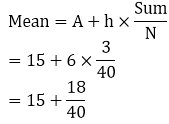= 15 + 0.45

= 15.47

Question: 6

Class interval:
50 - 70
70 - 90
90 - 110
110 - 130
130 - 150
150 - 170

Frequency:
18
12
13
27
8
22

Solution:

Let us assumed mean be 100

Class interval
Mid-value xi
di = xi – 100
ui = (xi – 100)/20
fi
fiui

50 - 70
60
-40
-2
18
-36

70 – 90
80
-20
-1
12
-12

90 – 110
100
0
0
13
0

110 – 130
120
20
1
27
27

130 – 150
140
40
2
8
16

150 – 170
160
60
3
22
66

N = 100
Sum = 61

A = 100, h = 20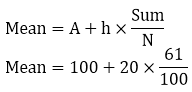= 100 + 12.2
= 122.2

Question: 7

Class interval:
0- 8
8 – 16
16 - 24
24 - 32
32 - 40

Frequency:
6
7
10
8
9

Solution:

Let the assumed mean (A) = 20

Class interval
Mid- value
di= xi - 20
ui = (xi – 20)/8
fi
fiui

0-8
4
-16
-2
6
-12

16-Aug
12
-8
-1
7
-7

16-24
20
0
0
10
0

24-32
28
8
1
8
8

32-40
36
16
2
9
18

N = 40
Sum = 7

We have A = 20, h = 8
Mean = A + h (sum/N)
= 20 + 8 (7/40)
= 20 + 1.4
= 21.4

Question: 8

Class interval:
0 - 6
6 – 12
12 – 18
18 - 24
24 - 30

Frequency:
7
5
10
12
6

Solution:

Let the assumed mean be (A) = 15

Class interval
Mid – value
di = xi -15
ui = (xi -15)/6
Frequency fi
fiui

0 – 6
3
-12
-2
-1
-14

6 – 12
9
-6
-1
5
-5

12 – 18
15
0
0
10
0

18 – 24
21
6
1
12
12

24 – 30
27
12
2
6
12

N = 40
Sum = 5

We have A = 15, h = 6
Mean = A + h(sum/N)
= 15 + 6 (5/40)
= 15 + 0.75
= 15.75

Question: 9

Class interval:
0 - 10
20 – 30
20 - 30
30 - 40
40 - 50

Frequency:
6
7
10
8
9

Solution:

Let the assumed mean (A) = 25

Class interval
Mid – value
di = xi -25
ui = (xi - 25)/10
Frequency fi
fiui

0 - 10
5
-20
-2
9
-18

20-Oct
15
-10
-1
10
-12

20 - 30
25
0
0
15
0

30 - 40
35
10
1
10
10

40 - 50
45
20
2
14
28

N = 60
Sum = 8

We have A = 25, h = 10
Mean = A + h (sum/N)
= 25 + 19 (8/60)
= 25 + (4/3)
= 26.333

Question: 10

Class interval:
0- 8
8 – 16
16 - 24
24 - 32
32 - 40

Frequency:
5
9
10
8
8

Solution:

Let the assumed mean (A) = 20

Class interval
Mid value xi
di= xi - 20
ui = (xi -20)/8
Frequency fi
fiui

0 – 8
4
-16
-2
5
-10

8 – 16
12
-8
-1
9
-9

16 – 24
20
0
0
10
0

24 – 32
28
8
1
8
8

32 – 40
36
16
2
8
16

N = 40
Sum = 5

We have, A = 20, h = 8
Mean = A + h (sum/N)
= 20 + 8 (5/ 40)
= 20 + 1
= 21

Question: 11

Class interval:
0- 8
8 – 16
16 - 24
24 - 32
32 - 40

Frequency:
5
6
4
3
2

Solution:

Let the assumed mean (A) = 20

Class interval
Mid value xi
di= xi - 20
ui = (xi -20)/8
Frequency fi
fiui

0 – 8
4
-16
-2
-2
-10

8  – 16
12
-8
-1
-1
-6

16 – 24
20
0
0
0
0

24 – 32
28
8
1
1
3

32 – 40
36
16
2
2
4

N = 20
Sum = -9

We have, A = 20, h = 8
Mean = A + h (sum/N)
= 20 + 8 (-9/ 20)
= 20 – (72/20)
= 20 – 3.6
= 16.4

Question: 12

Class interval:
20 – 30
30 - 50
50 - 70
70 - 90
90- 110
110 - 130

Frequency:
5
8
12
20
3
2

Solution:

Let the assumed mean (A) = 60

Class interval
Mid value xi
di= xi - 60
ui = (xi -60)/20
Frequency fi
fiui

10 – 30
20
-40
-2
5
-10

30 – 50
40
-20
-1
8
-8

50 – 70
60
0
0
12
0

70 – 90
80
20
1
20
20

90 – 110
100
40
2
3
6

110 – 130
120
60
3
2
6

N = 50
Sum = 14

We have A = 60, h = 20
Mean = A + h (sum/N)
= 60 + 20 (14/ 5)
= 60 + 5.6
= 65.6

Question: 13

Class interval:
25 - 35
35 – 45
45 - 55
55 - 65
65 - 75

Frequency:
6
10
8
10
4

Solution:

Let the assumed mean (A) = 50

Class interval
Mid value xi
di= xi - 50
ui = (xi - 50)/ 10
Frequency fi
fiui

25 – 35
30
-20
-2
6
-12

35 – 45
40
-10
-1
10
-10

45 – 55
50
0
0
8
0

55 – 65
60
10
1
12
12

65 – 75
70
20
2
4
8

N = 40
Sum = -2

We have A = 50, h = 10
Mean = A + h (sum/N)
= 50 + 10 (-2/ 40)
= 50 - 0.5
= 49.5

Question: 14

Class interval:
25 - 29
30 - 34
35 -39
40 - 44
45 - 49
50 - 54
55 - 59

Frequency:
14
22
16
6
5
3
4

Solution:

Let the assumed mean (A) = 42

Class interval
Mid value xi
di = xi - 42
ui = (xi - 42)/ 5
Frequency fi
fiui

25 – 29
27
-15
-3
14
-42

30 – 34
32
-10
-2
22
-44

35 – 39
37
-5
-1
16
-16

40 – 44
42
0
0
6
0

45 – 49
47
5
1
5
5

50 – 54
52
10
2
3
6

55 – 59
57
15
3
4
12

N = 70
Sum = -79

We have A = 42, h = 5
Mean = A + h (sum/N)
= 42 + 5 (-79/70)
= 42 – 79/14
= 36.357

Question: 15

For the following distribution, calculate mean using all suitable methods:

Size of item:
1 – 4
4 – 9
9 – 16
16 -20

Frequency:
6
12
26
20

Solution:

By direct method

Mean = (sum/N)
= 848/ 64= 13.25

By assuming mean method Let the assumed mean (A) = 65

Class interval
Mid value xi
ui = (xi - A) = xi - 65
Frequency fi
fiui

1 – 4
2.5
-4
6
-25

4 – 9
6.5
0
12
0

9 – 16
12.5
6
26
196

16 – 27
21.5
15
20
300

N = 64
Sum = 432

Mean = A + sum/N
= 6.5 + 6.75
= 13.25

Question: 16

The weekly observation on cost of living index in a certain city for the year 2004 – 2005 are given below. Compute the weekly cost of living index.

Cost of living index
Number of students
Cost of living index
Number of students

1400 – 1500
5
1700 – 1800
9

1500 – 1600
10
1800 – 1900
6

1600 – 1700
20
1900 – 2000
2

Solution:

Let the assumed mean (A) = 1650

Class interval
Mid value xi
di = xi – A = xi– 1650
ui = (xi – 1650)100
Frequency fi
fiui

1400 – 1500
1450
-200
-2
5
-10

1500 – 1600
1550
-100
-1
10
-10

1600 – 1700
1650
0
0
20
0

1700 – 1800
1750
100
1
9
9

1800 – 1900
1850
200
2
6
12

1900 – 2000
1950
300
3
2
6

N = 52
Sum = 7

We have A = 16, h = 100
Mean = A + h (sum/N)
= 1650 + 100 (7/52)
= 1650 + (175/13)
= 21625/13
= 1663.46

Question: 17

The following table shows the marks scored by 140 students in an examination of a certain paper:

Marks:
0 - 10
10 – 20
20 - 30
30 - 40
40 - 50

Number of students:
20
24
40
36
20

Solution:

(i) Direct method:

Class interval
Mid value xi
Frequency fi
fixi

0 – 10
5
20
100

10 – 20
15
24
360

20 – 30
25
40
1000

30 – 40
35
36
1260

40 – 50
45
20
900

N = 140
Sum = 3620

Mean = sum/ N
= 3620/ 140
= 25.857

(ii) Assumed mean method: Let the assumed mean = 25 Mean = A + (sum/ N)

Class interval
Mid value xi
ui = (xi - A)
Frequency fi
fiui

0 – 10
5
-20
20
-400

10 – 20
15
-10
24
-240

20 – 30
25
0
40
0

30 – 40
35
10
36
360

40 – 50
45
20
20
400

N = 140
Sum = 120

Mean = A + (sum/ N)
= 25 + (120/ 140)
= 25 + 0.857
= 25.857

(iii) Step deviation method: Let the assumed mean (A) = 25

Class interval
Mid value xi
di= xi – A = xi– 25
ui = (xi – 25)10
Frequency fi
fiui

0 – 10
5
-20
-2
20
-40

10 – 20
15
-10
-1
24
-24

20 – 30
25
0
0
40
0

30 – 40
35
10
1
36
36

40 – 50
45
20
2
20
40

N = 140
Sum = 12

Mean = A + h (sum/ N)
= 25 + 10(12/140)
= 25 + 0.857
= 25.857

Question: 18

The mean of the following frequency distribution is 62.8 and the sum of all the frequencies is 50. Compute the miss frequency f1 and f2.

Class:
0 - 20
20 – 40
40 - 60
60 - 80
80 - 100
100 - 120

Frequency:
5
f1
10
f2
7
8

Solution:

Class interval
Mid value xi
Frequency fi
fixi

0 – 20
10
5
50

20 – 40
30
f1
30f1

40 – 60
50
10
500

60 – 80
70
f2
70f2

80 – 100
90
7
630

100 – 120
110
8
880

N = 30 +fi + f2
Sum = 30f1+70 f2+ 2060

Given, sum of frequency
50 = f1 + f2 + 30
f1 + f2 = 50 – 30
f1 +f2 = 20
3f1 + 3f2 = 60 ---- (1)       [multiply both side by 3]
And mean = 62.8 Sum/ N
= 62.8 = (30f1+ 70f2+ 2060)/50
= 3140 = 30f1 + 70f2 + 2060
30f1+ 70f2 = 3140 – 2060
30f1 + 70f2 = 1080
3f1 + 7f2 = 108 ---- (2) [divide it by 10]
Subtract equation (1) from equation (2)
3f1 + 7f2 - 3f1 - 3f2 = 108 – 60
4f2 = 48 = f2 = 12

Put value of f2 in equation (1)
3f1 + 3(12) = 60
f1 = 24/3 = 8
f1 = 8, f2 = 12

Question: 19

The following distribution shows the daily pocket allowance given to the children of a multistory building. The average pocket allowance is Rs 18.00. Find out the missing frequency.

Class interval:
11 – 13
13- 15
15 - 17
17 - 19
19 - 21
21 - 23
23 - 25

Frequency:
7
6
9
13
-
5
4

Solution:

Given mean = 18, Let the missing frequency be v

Class interval
Mid value xi
Frequency fi
fixi

11 – 13
12
7
84

13 – 15
14
6
88

15 – 17
16
9
144

17 – 19
18
13
234

19 – 21
20
x
20x

21 – 23
22
5
110

23 – 25
14
4
56

N = 44 + x
Sum = 752 + 20x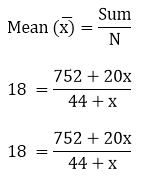792 + 18x = 752 + 20x
20x – 18x = 792 – 752
x = 40/2
x = 20

Question: 20

If the mean of the following distribution is 27. Find the value of p.

Class:
0 -10
10 – 20
20 - 30
30 - 40
40 - 50

Frequency:
8
p
12
13
10

Solution:

Class interval
Mid value xi
Frequency fi
fixi

0 – 10
5
8
40

10 – 20
15
P
152

20 – 30
25
12
300

30 – 40
35
13
455

40 – 50
45
16
450

N = 43 + P
Sum = 1245 + 15p

Given Mean = 27
Mean = sum/ N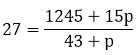1161 + 27p = 1245 + 15p
27p – 15p = 1245 – 1161
12p = 84
p = 84/12
p = 7

Question: 21

In a retail market, fruit vendors were selling mangoes kept in packing boxes. These boxes contain varying number of mangoes. The following was the distribution of mangoes according to the number of boxes.

Number of mangoes:
50 - 52
53 - 55
56 - 58
59 - 61
62 - 64

Number of boxes:
15
110
135
115
25

Find the mean number of mangoes kept in packing box. Which method of finding the mean did you choose?

Solution:

Number of mangoes
Number of boxes (fi)

50 – 52
15

53 – 55
110

56 – 58
135

59 – 61
115

62 – 64
25

We may observe that class internals are not continuous There is a gap between two class intervals. So we have to add 1/2 from lower class limit of each interval and class mark (xi) may be obtained by using the relation xi = (upper limit + lower class limit)/2. Class size (h) of this data = 3 Now taking 57 as assumed mean (a) we may calculated di , ui, fiui as follows

Class interval
Frequency fi
Mid value xi
di =  xi - A
ui = (xi – A)/3
fiui

49.5 – 52.5
15
51
-6
-2
-30

52.5 – 55.5
110
54
-3
-1
-110

55.5 – 58.5
135
57
0
0
0

58.5 – 61.5
115
60
3
1
115

61.5 – 64.5
25
63
6
2
50

Total
N = 400

Sum = 25

Now we have N = 400, Sum = 25,
Mean = A + h (sum/ N)
= 57 + 3 (25/400)
= 57 + 3/16
= 57+ 0.1875
= 57.19
Clearly mean number of mangoes kept in packing box is 57.19

Question: 22

The table below shows the daily expenditure on food of 25 households in a locality

Daily expenditure (in Rs):
100 - 150
150 - 200
200 - 250
250 -300
300 - 350

Number of households:
4
5
12
2
2

Find the mean daily expenditure on food by a suitable method.

Solution:

We may calculate class mark (xi) for each interval by using the relation xi = (upper limit + lower class limit)/2. Class size = 50 Now, talking 225 as assumed mean (xi) we may calculate di ,ui, fiui as follows:

Daily expenditure
Frequency fi
Mid value xi
di = xi ­– 225
ui = (xi – 225)50
fiui

100 – 150
4
125
-100
-2
-8

150 – 200
5
175
-50
-1
-5

200 – 250
12
225
0
0
0

250 – 300
2
275
50
1
2

300 – 350
2
325
100
2
4

N = 25

Sum = – 7

Now we may observe that N = 25 Sum = -7225 = 50 + (-7/ 25) × 225
225 – 14
= 211
So, mean daily expenditure on food is Rs 211

Question: 23

To find out the concentration of SO2 in the air (in parts per million i. e ppm) the data was collected for localities for 30 localities in a certain city and is presented below:

Concentration of SO2 (in ppm)
Frequency

0.00 – 0.04
4

0.04 – 0.08
9

0.08 – 0.12
9

0.12 – 0.16
2

0.16 – 0.20
4

0.20 – 0.24
2

Find the mean concentration of SO2 in the air

Solution:

We may find class marks for each interval by using the relation xi = (upper limit + lower class limit)/2. Class size of this data = 0.04 Now taking 0.04 assumed mean (xi) we may calculate di, ui, fiui as follows:

Concentration of SO2
Frequency fi
Class interval xi
di = xi – 0.14
ui
fiui

0.00 – 0.04
4
0.02
-0.12
-3
-12

0.04 – 0.08
9
0.06
-0.08
-2
-18

0.08 – 0.12
9
0.1
-0.04
-1
-9

0.12 – 0.16
2
0.14
0
0
0

0.16 – 0.20
4
0.18
0.04
1
4

0.20 – 0.24
2
0.22
0.08
2
4

Total
N = 30

Sum = -31

From the table we may observe that N = 30, Sum = - 31= 0.04 + (-31/30) × (0.04)
= 0.099 ppm
So mean concentration of SO2 ¬in the air is 0.099 ppm

Question: 24

A class teacher has the following absentee record of 40 students of a class for the whole term. Find the mean number of days a student was absent.

Number of days:
0 - 6
6 – 10
10 – 14
14 - 20
20 - 28
28 - 38
38 - 40

Number of students:
11
10
7
4
4
3
1

Solution:

We may find class mark of each interval by using the relation xi = (upper limit + lower class limit)/2 Now, taking 16 as assumed mean (a) we may Calculate di and fi di  as follows

Number of days
Number of students fi
Xi
d = xi - 16
fidi

0 – 6
11
3
-13
-143

6 – 10
10
8
-8
-80

10 – 14
7
12
-4
-28

14 – 20
7
16
0
0

20 – 28
8
24
8
64

28 – 36
3
33
17
51

30 – 40
1
39
23
23

Total
N = 40

Sum = -113

Now we may observe that N = 40, Sum = -11316 + (-113/ 40)
= 16 – 2.825
= 13.75
So mean number of days is 13.75 days, for which student was absent

Question: 25

The following table gives the literacy rate (in percentage) of 35 cities. Find the mean literacy rate.

Literacy rate (in %):
45 -55
55 - 65
65 - 75
75 - 85
85 - 95

Number of cites:
3
10
11
8
3

Solution:

We may find class marks by using the relation xi = (upper limit + lower class limit)/2 Class size (h) for this data = 10 Now taking 70 as assumed mean (a) wrong Calculate di ,ui, fiui as follows

Literacy rate (in %)
Number of cities (fi)
Mid value xi
di= xi ­– 70
ui = (di –A)10
fiui

45 – 55
3
50
-20
-2
- 6

55 – 65
10
60
-10
-1
-10

65 – 75
11
70
0
0
0

75 – 85
8
80
10
1
8

85 – 95
3
90
20
2
6

Total
N = 35

Sum = -2

Now we may observe that N = 35, Sum = -2= 70 + (-2/35) × 10
= 70 – 4/7
= 70 – 0.57
= 69.43
So, mean literacy rate is 69.43 %
```### Course Features

• 728 Video Lectures
• Revision Notes
• Previous Year Papers
• Mind Map
• Study Planner
• NCERT Solutions
• Discussion Forum
• Test paper with Video Solution